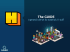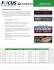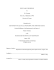# Point Sample Rendering J.P. Grossman/ W. Dally Alexandre Desboeufs An article by

## Transcription

Point Sample Rendering J.P. Grossman/ W. Dally Alexandre Desboeufs An article by
```Point Sample Rendering
An article by
J.P. Grossman/ W. Dally
presented by
Alexandre Desboeufs
Contents
1. Introduction
2. Object Sampling
2.1
2.2
2.3
2.4
2.5
Mesh Of Points
Memory Structure
The Algorithm
Magnification / Perspective
3. Rendering
3.1
3.2
3.3
3.4
3.5
Visibility Testing
Block Warping
Finding Gaps
4. Conclusion
2
1. Introduction
In Point Sample Rendering, objects are modeled as a dense set of surface point samples. These samples are
obtained from orthographic views and are stored with colour, depth and normal information, enabling Z-buffer
composition, Phong shading, and other effects such as shadows. Motivated by image based rendering, point
sample rendering is similar in that it also takes as input existing views of an object and then uses these to
synthesize novel views. It is different in that (1) the point samples contain more geometric information than image
pixels, and (2) these samples are view independent - that is, the colour of a sample does not depend on the direction
point sample representation
object
Figure 2.1: Point sample representation of an object.
from which it was obtained.
The use of points as a rendering primitive is appealing due to the speed and simplicity of such an approach.
Points can be rendered extremely quickly; there is no need for polygon clipping, scan conversion, texture
mapping or bump mapping. Like image based rendering, point sample rendering makes use of today’s
large memories to represent complex objects in a manner that avoids the large render-time computational
costs of polygons. Unlike image based representations, which are view dependent and will therefore
sample the same surface element multiple times, point sample representations contain very little
redundancy, allowing memory to be used efficiently.
2. Object Sampling
2.1
Mesh of Points
One approach to the problem of surface reconstruction is to treat the point samples as vertices of a
triangular mesh which can be scan-converted. We have rejected this approach for a number of reasons.
First and foremost, it is slow, requiring a large number of operations per point sample. Second, it is
difficult to correctly generate the mesh without some a priori knowledge of the object’s surface topology;
there is no exact way to determine which points should be connected to form triangles and which points lie
on different surfaces and should remain unconnected. Invariably one must rely on some heuristic which
compares depths and normals of adjacent points. Third, it is extremely difficult to ascertain whether or not
the entire surface of the object is covered by the union of all triangles from all views, especially if we are
retaining only a subset of the point samples from each view. Fourth, when a concave surface is sampled by
multiple views, the triangles formed by points in one view can obscure point samples from other views
(figure 2.2a). This degrades the quality of rendered images by causing pixels to be filled using the less
accurate colour obtained from triangle interpolation rather than the more accurate point sample which lies
behind the triangle. Fifth, noticeable artifacts result when we attempt to combine triangles from multiple
views in a single image. Far from merging seamlessly to form a single continuous surface, triangles from
different views appear incoherent, as shown in figure 2.2b.
3
(a)
(b)
(c)
Figure 2.2
Another approach is the use of ‘splatting’, whereby a single point is mapped to multiple pixels on the
screen, and the colour of a pixel is the weighted average of the colours of the contributing points.
However, this is again slow, requiring a large number of operations per point. Furthermore, choosing the
size and shape of the splat to ensure that there are no tears in any surface is an extremely difficult problem.
One interesting idea is to use point sample normals to draw small oriented circles or quadrilaterals, but this
results in ‘overshoot’near corners as shown in figure 2.2c.
2.2
In order to maximize speed, our solution essentially ignores the problem altogether at render. To see how
this can be achieved without introducing holes into the image, we start with the simplifying assumptions
that the object will be viewed orthographically, and that the target resolution and magnification at which it
will be viewed are known in advance. We can then, in principle, choose a set of surface point samples
which are dense enough so that when the object is viewed there will be no holes, independent of the
viewing angle. We say that an object or surface is adequately sampled (at the given resolution and
magnification) if this condition is met.
pixel
pixel contained
point
pixel hit
ds
Figure 2.3
We begin by establishing a geometric condition which guarantees that a set of point samples forms an
adequate sampling of a surface. Suppose we overlay a finite triangular mesh on top of a regular array of
square pixels. We say that a pixel is contained in the triangular mesh if its center lies within one of the
triangles (figure 2.3). As before, we say that a pixel is hit by a mesh point if the mesh point lies inside the
pixel.
Theorem If the side length of each triangle in the mesh is less than the pixel side length, then every pixel
which is contained in the mesh is hit by some point in the mesh.
Corollary Suppose a surface is sampled at points which form a continuous triangular mesh on the surface.
If the side length of every triangle in the mesh is less than the side length of a pixel at the target resolution
(assuming unit magnification), then the surface is adequately sampled.
In particular, it suggests that to minimize the number of samples, the distance between adjacent samples on
the surface of the object should be as large as possible but less than ds, the pixel side length at the target
resolution (again assuming unit magnification). In order to obtain such a uniform sampling, we sample
orthographic views of the object on an equilateral triangle lattice. Now it is possible for the distance
between adjacent samples on the object to be arbitrarily large on surfaces which are nearly parallel to the
viewing direction (figure 2.4a). However, suppose we restrict our attention to a surface (or partial surface)
S whose normal differs by no more than θ from the projection direction at any point, where θ is a ‘tolerance
angle’. If we sample the orthographic view on an equilateral triangle lattice with side length ds·cosθ, we
4
obtain a continuous triangular mesh of samples on S in which each side length is at most (ds·cosθ) / cosθ =
ds (figure 2.4b). Hence, S is adequately sampled.
Rather than sampling the orthographic projections on a square lattice at the target resolution, we sample on
a denser equilateral triangle lattice. The density of the lattice is determined by the tolerance angle; if the
tolerance angle is θ then the spacing between samples is ds·cosθ (where ds is the pixel side length), which
gives us 2
samples per pixel. We typically use θ = 25° which translates to 1.4 samples per
3 cos 2 θ
pixel.
ds
θ
θ
ds·cos
θ
viewing
direction
(a)
(b)
Figure 2.4
2.3
Memory Structure
Each point sample contains a depth, an object space surface normal, diffuse colour, specular colour and
shininess. Table 2.5 gives the amount of storage associated with each of these; an entire point will fit into
three 32 bit words. Surface normals are quantized to one of 32768 unit vectors (figure 2.6). These vectors
are generated using the same procedure described previously to generate projection directions, except that
there are 6 levels of subdivision rather than 1, producing 32768 spherical triangles. The vectors are stored
in a large lookup table which is accessed when the points are shaded at render time.
Field
# bits
Description
Depth
32
Single precision floating point
Object space normal
15
quantized to one of 32768 vectors
Diffuse colour
24
8 bits of red, green, blue
Specular colour
16
6 bits of green, 5 bits of red, blue
Shininess
8
Total
95
Table 2.5: Data associated with each point sample
Figure 2.6: The sphere of directions is subdivided into 128 triangles. Each triangle contains a set
of directions which is represented by a single bit in the visibility mask.
5
The samples in a projection are divided into 8x8 blocks. This allows us to
compress the database by retaining only those blocks which are needed while
maintaining the rendering speed and storage efficiency afforded by a regular
lattice (a regular lattice can be rendered quickly by using incremental
calculations to transform the points, and it can be stored more compactly than
a set of unrelated points as we only need to store one spatial coordinate explicitly). In addition,
the use of blocks makes it possible to amortize visibility tests over groups of 64 points.
2.4
The Algorithm
The union of point samples from all projections is assumed to form an adequate sampling of the object.
The next task in the modeling process is to find a subset S of blocks which is still an adequate sampling but
contains as little redundancy as possible. For this we use a greedy algorithm.
We start with no blocks (S = φ). We then step through the list of orthographic projections; from each
projection we select blocks to add to S. A block is selected if it corresponds to a part of the object which is
not yet adequately sampled by S. Rather than attempt to exactly determine which surfaces are not
adequately sampled, which is extremely difficult, we employ the heuristic of both ray tracing the
orthographic projection and reconstructing it from S, then comparing the depths of the two images thus
obtained (figure 2.7). This tests for undersampled surfaces by searching for pixels which are ‘missed’ by
the reconstruction. If a pixel is missing from a surface then, by definition, that surface is not adequately
sampled. However, if no pixels are missing from a surface it does not follow that the surface must be
adequately sampled, as there may be some other rotated/translated view in which the surface will contain a
hole. Thus, to enhance the quality of the test it is repeated several times using various translations and
rotations for the view. Typically, we use every combination of four rotations (0°, 22.5°, 45°, 67.5°) and
four translations (either no offset or a one half pixel offset in both x and y), a total of sixteen tests.
(a)
(b)
(c)
(d)
Figure 2.7: Block Selection. (a) Ray traced projection. (b) Projection reconstructed from current set of
blocks. (c) Pixels where (a) and (b) have different depths. (d) Blocks added to set.
Since we start with no blocks, there will be a bias towards including blocks from earlier projections.
Indeed, for the first two projections, which are taken from opposite directions, all the blocks which contain
samples of the object will necessarily be included. To eliminate this bias we make a second pass through
the projections in the same order; for each projection we remove from S any of its blocks that are no longer
needed. This second pass has been observed to decrease the total number of blocks in the set by an average
of 22%.
2.5
Magnification / Perspective
The concept of an ‘adequately sampled’ surface does not solve the surface reconstruction problem; if we
wish to magnify the object we are once again confronted by the original dilemma. However, suppose that
the object is adequately sampled at the original resolution/magnification, and suppose we decrease the
image resolution by the same amount that the magnification is increased. The object will again be
adequately sampled at this new resolution/magnification. Thus, we can render the object at the lower
resolution and resample the resulting image to the desired resolution.
This works well for reconstructing orthographic views of the object, but when we try to adapt the solution
to perspective views we encounter a problem: because of the perspective transform, different parts of the
object will be magnified by different amounts. A straightforward solution would be to choose a single
lower resolution taking into account the worst case magnification of the object, i.e. the magnification of the
6
part closest to the viewer. However, this unfairly penalizes those parts of the object which would be
adequately sampled at a higher resolution, and it unnecessarily degrades the quality of the final image.
Figure 2.8: The dark surface is rendered using a higher level depth buffer in which there are no gaps. This
depth buffer is used to detect and eliminate holes in the image.
To address this problem, we introduce a hierarchy of lower resolution depth buffers as in [Green93]. The
lowest buffer in the hierarchy has the same resolution as the target image, and each buffer in the hierarchy
has one half the resolution of the buffer below. Then, in addition to mapping point samples into the
destination image as usual, each part of the object is rendered in a depth buffer at a low enough resolution
such that the points cannot spread out and leave holes. When all parts of the object have been rendered, we
will have an image which, in general, will contain many gaps. However, it is now possible to detect and
eliminate these holes by comparing the depths in the image to those in the hierarchy of depth buffers (figure
2.8). The next two sections provide details of this process.
3. Rendering
3.1
Visibility Testing
The first method which is used to test a block’s visibility is to see if it lies within the view frustum – the
region of space that is in the camera’s field of view. As usual, the view frustum is defined by a ‘near’
plane, a ‘far’ plane, and the four planes which pass through the camera and the sides of the screen (figure
3.1). We use a block’s bounding sphere to determine whether or not the block lies entirely outside one of
these planes. If it does, then we don’t need to render it.
If a block lies inside the view frustum, then whether or not it is visible depends on whether or not it is
obscured by some other part of the object, which in turn depends on the position of the camera. A brute
force approach to determining visibility, then, is to subdivide space into a finite number of regions and to
associate with each region a list of potentially visible blocks. However, we chose not to implement a
visibility test based on spatial subdivision due to the large amount of storage required. Since this is a three
dimensional problem, the number of bits of storage which are needed is proportional to the number of
blocks times the cube of the resolution of the spatial subdivision.
near plane
far plane
Figure 3.1: View Frustum
One way to lessen the amount of storage required is to reduce the dimensionality of the problem. If we
restrict our attention to viewpoints which lie outside the convex hull of the object, then whether or not a
block is visible depends only on the direction from which it is viewed. Visibility as a function of direction
is a two dimensional problem which we tackle using visibility masks.
7
To compute the visibility masks, we render the entire set of blocks orthographically from a number of
directions in each triangle (typically ten directions per triangle). Each point is tagged with a pointer to its
block; this tag is used to determine the subset of blocks which contribute to the orthographic images. The
visibility masks in this subset of blocks are updated by setting the appropriate triangle bit.
The simplest way to make use of these masks at render time is to construct a visibility mask for the screen.
In this mask, the kth bit is set if and only if some direction from a screen pixel to the eye lies inside the kth
triangle on the triangulated sphere of directions. We then AND this mask with each block’s mask; a block
is definitely not visible if the result of this test is zero (illustrated in two dimensions in figure 3.2).
0
0
0
0
0
0
1
0
1
1
1
1
0
0
1
1
0
0
0
0
0
0
(a)
1
(b)
0
0
0
0
0
0
(c)
0
0
0
(d)
Figure 3.2: Using visibility masks to test visibility. (a) Eye-screen directions. (b) Set of directions
from which a point is visible. (c) Screen mask generated from set of screen-eye directions. (d)
Visibility mask of point. We can deduce that the point is not visible from the fact that the AND of the
One of the basic visibility tests used in polygon rendering is “backface culling”; there is no need to render
polygons whose normals are pointing away from the viewer. We can adapt this test to point sample
rendering. We begin with the observation that, similar to polygons, the tangent plane at each point sample
defines a half space from which the point is never visible. Taking the intersection of these half spaces over
all the points in a block, we obtain a region in space, bounded by up to 64 planes, from which no part of the
block is ever visible. It would be quite expensive to attempt to exactly determine whether or not the camera
lies inside this region. Instead, we can approximate the region by placing a cone inside it. This visibility
cone gives us a fast, conservative visibility test; no part of the block is visible from any viewpoint within
the cone.
(a)
(b)
(c)
Figure 3.3: Visibility cone in two dimensions. (a) Half space from which point is never
visible. (b) Region from which no part of the block is visible. (c) Cone inside region.
3.2
Block Warping
In this section we will describe the basic Point Sample Rendering algorithm which maps each point sample
to the nearest pixel in the destination image. We will show how the computations can be optimized using
incremental calculations. We ignore for the time being the hierarchical Z-buffer which will be discussed in
section chapter 2.5.
Recall that the points in a block are stored in the coordinates of the orthographic projection from which the
block was obtained. We begin, then, by computing, for each projection, the transformation from projection
space to camera space. By camera space we mean the coordinate system in which the camera is at the
origin and the screen is a square of side 2 centered and axis-aligned in the plane z = 1. We can write this
transformation as
8
Axx Axy Axz x  v x 
x' 
x 

   
 
 
y' = A y  + v = Ayx Ayy Ayz y + v y 
Azx Azy Azz z  v z 


z ' 

z 


   
where (x, y, z) are the projection space coordinates of a point, ( x’, y’, z’) are the camera space coordinates,
A is an orthogonal matrix giving the rotation and scale components of the transformation, and v is a
translation vector. We can then compute the integer screen coordinates ( u, v) from the camera space
coordinates with a perspective divide and a scale/offset which maps [-1, 1] to [0, N] as follows:
N  y' 
N  x' 
u =   + 1
v =   + 1
 2  z ' 
 2  z ' 
In order to implement the surface reconstruction algorithm presented in chapter 2.5, each block must be
warped into the hierarchy of Z-buffers at a low enough resolution such that its points can’t spread out and
N  N 
leave holes. If the image resolution is NxN, then the kth depth buffer has resolution  k × k  where ·
2  2 
denotes the least integer function. To select an appropriate depth buffer for the block, we start with the
assumption that the block is an adequate sampling for orthographic views with unit magnification at MxM
pixels. Suppose that the object is being rendered with magnification γ
, and let d = min z i ' where zi ' is the
i
camera space depth of the ith point in the block as before. Then the worst case effective magnification of
the block, taking into account the change in resolution, the object magnification, and the perspective
projection, is
γ
N
Md
To compensate for this, we need to choose the depth buffer such that
Thus, we take
γ
N
Md 2 k
≤1
  γ
N 
k = max0, log


Md





It is fairly expensive to compute d = min z i ' exactly.
As an approximation, we can instead use the
i
distance d’from the camera to the bounding sphere of the block. This provides a lower bound for d and is
much easier to calculate.
If k = 0, then the block is being warped into the usual depth buffer. If k > 0, then we need to augment the
warping procedure as follows:
for each point
compute u, v, z’
if (z’ < depth(0, u, v))
depth(0, u, v) = z’
copy point to pixel(u, v)
u’ = u/2^k
v’ = v/2^k
if (z’ + threshold < depth(k, u’, v’))
depth(k, u’, v’) = z’ + threshold
end if
end for
where depth(k, u, v) denotes the kth Z-buffer depth at (u, v).
Note that instead of simply testing the point’s Z value against the value in the depth buffer, we first add a
small threshold. The reason for this is that the depth buffer will be used to filter out points which lie behind
the foreground surface; the use of a threshold prevents points which lie on the foreground surface from
being accidentally discarded. For example, suppose 3 points from a foreground surface and 1 point from a
background surface are mapped to four different image pixels contained in a single depth map pixel (shown
in one dimension in figure 3.4). Without the threshold, all but the nearest of these points will be discarded
(figure 3.4a). The use of a threshold prevents this from happening (figure 3.4b), allowing us to use all or
most of the surface points when computing the final image. We found a good choice for the threshold to be
9
three times the image pixel width; smaller thresholds produce images of lower quality, while larger
thresholds begin to have difficulty discriminating between foreground and background surfaces. Figure 3.5
shows the striking difference in image quality which results from the use of a threshold; the left image was
rendered using a threshold of three times the image pixel width.
Z
Z
(a)
(b)
Figure 3.4: Four points are mapped to different image pixels contained in a single depth map pixel. (a) No
depth threshold - only the nearest point is retained. (b) The use of a small threshold prevents points on the
same surface as the nearest point from being discarded
Figure 3.5: Depth no threshold (left) vs. Threshold (right).
3.3
Finding Gaps
The easiest way to use the hierarchical depth buffer to detect holes is to treat a pixel in the kth depth buffer
as an opaque square which covers exactly 4 k image pixels. We can then make a binary decision for each
pixel by determining whether or not it lies behind some depth buffer pixel. If a pixel is covered by a depth
buffer pixel, then we assume that it does not lie on the foreground surface, i.e. it is a hole. In the final
image this pixel must be recoloured by interpolating its surroundings. If a pixel is not covered by any depth
buffer pixels, then we assume that it does lie on the foreground surface, and its colour is unchanged in the
final image. This method is fast and easy to implement. However, it produces pronounced blocky artifacts,
particularly at edges, as can be seen in figure 3.6-up.
Our strategy for dealing with this problem is to replace the binary decision with a continuous one. Each
pixel will be assigned a weight in [0, 1] indicating a ‘confidence’ in the pixel, where a 1 indicates that the
pixel is definitely in the foreground and a 0 indicates that the pixel definitely represents a hole and its
colour should be ignored. The weights will then be used to blend between a pixel’s original and
interpolated colours. To see how these weights can be obtained, we observe that: (i) if a pixel is
surrounded by depth buffer pixels which are closer to the viewer, then it is certainly a hole and should have
weight 0; (ii) if a pixel is surrounded by depth buffer pixels which are further away from the viewer, then it
is certainly in the foreground and should have weight 1; (iii) if a pixel is surrounded by depth buffer pixels
both closer to and further away from the viewer, then it lies near the edge of the surface and should have a
weight between 0 and 1. It therefore makes sense to consider multiple depth buffer pixels when assigning a
weight to a single image pixel.
In figure 3.6-down shows the weights that are assigned to image pixels using bilinear interpolation. These
weights are used to blend between each pixel’s original and interpolated colours; in the final image the
artifacts are much less prominent (3.6-up).
10
yes /no
bilinear
Figure 3.6: (up) the simple method, (down) the bilinear method
3.4
Shadows are computed using shadow maps. For each light, we render the object from the light’s viewpoint
and use a hierarchical depth map to store Z values. Since we are only interested in creating a depth map,
we don’t have to worry about copying points’ reflectance information into a destination image. The
warping procedure is thus greatly simplified. For each block we again choose an appropriate level k in the
depth buffer hierarchy and then warp the points as follows:
for each point
compute u’, v’, z’
if (z’ < depth(k, u’, v’))
depth(k, u’, v’) = z’
end if
end for
To calculate how much of a given point is in shadow, we first transform the point to light space, the
coordinate system of the light’s hierarchical depth map. We then compute a coverage for the point using
almost exactly the same procedure as that used in section 3.3 for detecting gaps. The only difference is that
we subtract a small bias from the point’s depth before comparing it to the depths in the shadow map, to
prevent surfaces from casting shadows on themselves due to small inaccuracies in the computation of the
points’light space coordinates. In our case, these inaccuracies arise primarily from the fact that the points’
x’and y’camera space coordinates are rounded to pixel centers.
Since the warping procedure used to create shadow maps is so simple, it is extremely fast. As a result,
shadows can be computed with much less overhead than would normally be expected from an algorithm
which re-renders the object for each light source. For example, figure 3.7 was rendered at 256x256 pixels
with three light sources - two directional lights and a spotlight. It took 204ms to render without shadows
and 356ms with shadows - an overhead of less than 25% per light source.
11
3.5
To fill the gaps in the final image we must compute a colour for pixels with weight less than 1. This
problem is not specific to point sample rendering; it is the general problem of image reconstruction given
incomplete information. In the ‘pull’phase we compute a succession of lower resolution approximations of
the image, each one having half the resolution of the previous one. In the ‘push’phase, we use these lower
resolution images to fill gaps in the higher resolution images. This is illustrated in figure 3.8 with a picture
of a mandrill.
(a)
(b)
(c)
(d)
Figure 3.8: The pull-push algorithm. (a) Original image. (b) Incomplete image; 50% of the pixels have
been discarded. (c) Lower resolution approximations. (d) Reconstruction.
The lower resolution approximations of the image are computed by repeatedly averaging 2x2 blocks of
pixels, taking the weight of the new pixel to be the sum of the weights of the four pixels, capped at 1.
Specifically, if cxk, y and w xk, y are the colour and weight of the (x, y) pixel in the kth low resolution
approximation, then:
c xk,+y1 =
w 2k x, 2 y c 2k x ,2 y + w 2k x+ 1, 2 y c 2k x + 1,2 y + w 2k x,2 y + 1c 2k x, 2 y + 1 + w 2k x + 1, 2 y + 1 c 2k x + 1,2 y + 1
w 2k x ,2 y + w 2k x+ 1, 2 y + w 2k x, 2 y + 1 + w 2k x + 1, 2 y + 1
w xk,+y1 = MIN (1, w 2k x, 2 y + w 2k x + 1,2 y + w 2k x,2 y + 1 + w 2k x + 1, 2 y + 1 )
k
th
In the ‘push’phase, to compute the final colour c′
x , y of the (x, y) pixel in the k low resolution image, we
k
k
inspect its weight w xk, y . If w xk, y = 1 then we simply set c ′
x, y = c x, y . Otherwise, we compute an interpolated
colour c~ k using as interpolants the pixels in the ( k+1)st image. We then set
x, y
k
k
k
k
~k
c′
x , y = w x, y c x , y + (1 − w x, y ) c x, y
If we were to use bilinear interpolation to compute c~xk, y , then we would interpolate the four closest pixels
in the (k+1)st image with weights 9/16, 3/16, 3/16 and 1/16 (since the interpolation is always from one level
up in the hierarchy, the weights never change). However, if we approximate these weights as ½, ¼, ¼ and
0, then the algorithm runs significantly faster (over 6 times faster using packed RGB arithmetic; see
Appendix) without any noticeable degradation in image quality. Hence, we interpolate the three closest
pixels in the (k+1)st image with weights ½, ¼ and ¼. For example,
1 k+ 1 1 k+ 1 1 k+ 1
c~2kx + 1, 2 y + 1 = c ′
c′
c′
x, y +
x + 1, y +
x, y + 1
2
4
4
with similar expressions for c~2kx, 2 y , c~2kx + 1,2 y , and c~2kx, 2 y + 1 .
12
3.6
Filing Gaps
After all the blocks have been warped and weights have been assigned to image pixels as described in
section 3.3, the non-background pixels with positive weight must be shaded according to the current
lighting model. Performing shading in screen space after pixels have been weighted saves time by shading
only those points which contribute to the final image. For our purposes the lighting model is Phong
shading with shadows, but one could certainly implement a model which is more or less sophisticated as
needed.
Since point sample normals are stored in object space, we perform shading calculations in object space to
avoid transforming the normals to another coordinate system. We therefore need to recover object space
coordinates for the points contained in the image pixels. Since we do not store the exact camera space x’
and y’coordinates for points when they are copied to pixels in the image buffer, we assume that they are
located at the pixel centers. Using the z’coordinates which are stored in the image Z-buffer, we can then
transform the points from camera space to object space. The pixel centers form a regular square lattice, so
this transformation can be done quickly using incremental calculations.
It is worth noting that because of the dense reflectance information contained in point sample models, the
resulting specular highlights are of ray-trace quality, as can be seen in figure 3.9.
Figure 3.9: High quality specular highlights.
4. Conclusion
Point Sample Rendering is an algorithm which features the speed of image based graphics with quality,
flexibility and memory requirements approaching those of traditional polygon graphics. Objects are
represented as a dense set of point samples which can be rendered extremely quickly. These point samples
contain precise depth and reflectance information, allowing objects to be rendered in dynamically lit
environments with no geometric artifacts. Because the point samples are view-independent, point sample
representations contain very little redundancy, allowing memory to be used efficiently.
A novel solution to the problem of surface reconstruction has been introduced, using a combination of
adequate sampling and hierarchical Z-buffers to detect and repair surface tears. This approach does not
sacrifice the speed of the basic rendering algorithm, and in certain cases it guarantees that images will not
contain holes. It also been shown how a variant of percentage closer filtering can be used to eliminate
artifacts which result from a straightforward utilization of the hierarchical Z-buffer.
Because of its flexibility, Point Sample Rendering is suitable for modeling and rendering complex objects
in virtual environments with dynamic lighting such as flight simulators, virtual museums and video games.
It is also appropriate for modeling real objects when dense reflectance information is available. Since Point
Sample Rendering has low memory requirements, it can be implemented on inexpensive personal
computers.
The authors have shown that it is possible to render directly from point samples. The software system,
implemented on a 333MHz Pentium II, has demonstrated the ability of Point Sample Rendering to render
complex objects in real time. It has also shown that the resulting images are of high quality, with good
shadows and ray-trace quality specular highlights.
13
Dragon
File Size (in mega byte))
Number of blocks
Construction time (h : m)
Kittyhawk
Toybird
Pine Tree
Dragon
Kittyhawk
Toybird
Pine Tree
0.96
2100
00:18
1.73
3390
01:02
1.46
2411
00:11
7:12
11051
01:35
10
5
62
8
23
7
3
118
9
9
226
4
25
7
2
282
10
4
50
8
23
7
3
105
Rendering time (in ms on a 333 Mhz Pentium II)
Init
10
Visibility
3
Warp
36
Find gaps
7
18
Pull
7
Push
3
Total
84
14
```

### NIS-Elements: How to use Pixel Classifier to produce an Area Fraction... Pixel Classifier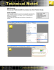### XMPlayer Express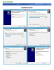### Pixel Perfect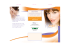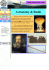### Point Sample Rendering J.P. Grossman and William J. Dally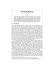### Good Morning/Afternoon, my name is Michael - Light-O-Rama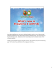### optoSURF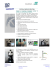### Perfect Pixel Implementation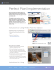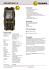### The GUIDE